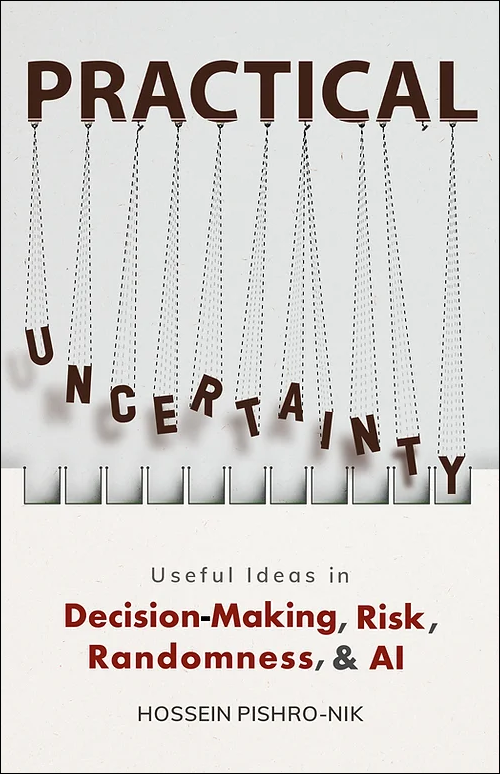## 3.1.2 Discrete Random Variables

There are two important classes of random variables that we discuss in this book: discrete random variables and continuous random variables. We will discuss discrete random variables in this chapter and continuous random variables in Chapter 4. There will be a third class of random variables that are called mixed random variables. Mixed random variables, as the name suggests, can be thought of as mixture of discrete and continuous random variables. We will discuss mixed random variables in Chapter 4 as well.

Remember that a set $A$ is countable if either

• $A$ is a finite set such as $\{1,2,3,4\}$, or
• it can be put in one-to-one correspondence with natural numbers (in this case the set is said to be countably infinite)
In particular, as we discussed in Chapter 1, sets such as $\mathbb{N}, \mathbb{Z}, \mathbb{Q}$ and their subsets are countable, while sets such as nonempty intervals $[a,b]$ in $\mathbb{R}$ are uncountable. A random variable is discrete if its range is a countable set. In Example 3.2, the random variables $X$ and $Y$ are discrete, while the random variable $T$ is not discrete.

$X$ is a discrete random variable, if its range is countable.

 The print version of the book is available on Amazon.Practical uncertainty: Userful Ideas in Decision-Making, Risk, Randomness, & AI